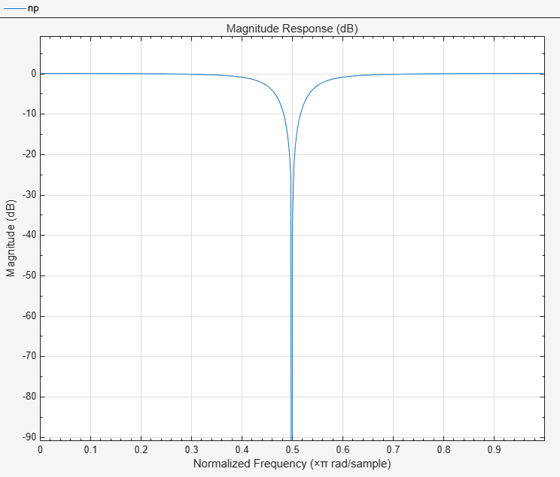# getOctaveBandwidth

Bandwidth in number of octaves

## Syntax

``N = getOctaveBandwidth(npFilter)``

## Description

example

````N = getOctaveBandwidth(npFilter)` returns the bandwidth of the notch peak filter, measured in number of octaves.```

## Examples

collapse all

Create a `dsp.NotchPeakFilter` object in the default configuration.

`np = dsp.NotchPeakFilter`
```np = dsp.NotchPeakFilter with properties: Specification: 'Bandwidth and center frequency' Bandwidth: 2205 CenterFrequency: 11025 SampleRate: 44100 ```

Determine the octave bandwidth of the filter using the `getOctaveBandwidth` function.

`getOctaveBandwidth(np)`
```ans = 0.2881 ```

Visualize the filter response using `fvtool`.

`fvtool(np)`## Input Arguments

collapse all

Notch peak filter whose bandwidth is measured in octaves, specified as a `dsp.NotchPeakFilter` object.

## Output Arguments

collapse all

Bandwidth of the filter measured in number of octaves, returned as a scalar.

Data Types: `double`

### Objects

Introduced in R2014a

## SupportGet trial now